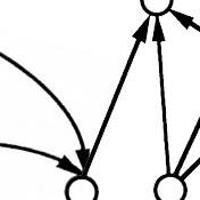## Histograms with functions of OpenCV

If something is useful for computer vision, you'll almost definitely find it in OpenCV. Histograms are no exception. And OpenCV comes with a rich set of functions to work with and manipulate histograms.

## Creating and Releasing

Histograms are stored in the CvHistogram data structure:

```typedef struct CvHistogram
{
int type;
CvArr* bins;
float thresh[CV_MAX_DIM];   // for uniform histograms
float** thresh2;               // for nonuniform histograms
CvMatND mat;                   // embedded matrix header for array histograms
}
CvHistogram;
```

To create a new histogram, use the cvCreateHist function:

```CvHistogram* cvCreateHist(int dims,
int* sizes,
int type,
float** ranges = NULL,
int uniform = 1
);
```

Very simple to understand. Here's what the parameters mean:

• dims: The number of dimensions
• *sizes: This is an array with the same number of integers as the number of dimensions. Each integer specifies how many bins are created in each dimension.
• type: Possible values include CV_HIST_SPARSE or CV_HIST_ARRAY (usually, you'll want this one)
• **ranges: A two dimensional array. It's actual value depends on the next parameter, uniform
• If uniform=1, then you need to supply two floating point numbers per dimension. They are the starting and ending values of the range. Each bin is assigned a range based on these two values. (if you have 10 bins and you supply 0.0 and 10.0 in ranges, they you'll have each bin correspond to 0.0…0.99, 1…1.99, 2…2.99, etc. The "subdivisions" are calculated automatically)
• If uniform=0, then you need to supply N+1 floating point numbers per dimension. These are the starting and ending value for each bin. (If you have 10 bins, then you must supply 11 numbers, say a,b,c,d,e,f,g,h,I,j,k. Then bins correspond to a…b, b…c, c…d, etc)
• Uniform: If uniform is set to zero, the histogram is made non-uniform. For any non-zero value, the histogram is uniform. In the cvCreateHist function, you can actually set ranges to NULL. But then you'll have to set the ranges later on. You must do this with:

:::c++ void cvSetHistBinRanges(CvHistogram hist, float* ranges, int uniform = 1 );

The function just sets the ranges for the histogram hist. The parameters work just as explained above.

Once you're done with a histogram, it's a good idea to release it from memory. Other programs could really use some extra free RAM. You do this with:

```void cvReleaseHist(CvHistogram** hist);
```

This is similar to most other cvRelease type commands.

## Utility functions

### Reusing histograms

If you've already made a histogram and want to reuse it, you need to set each of the bins to zero. This is done by "clearing" the histogram:

```void cvClearHist(CvHistogram* hist);
```

If you already have an array and you want to convert it into a histogram, there's another utility function.

```CvHistogram* cvMakeHistHeaderForArray(int dims,
int* sizes,
CvHistogram* hist,
float* data,
float** ranges = NULL,
int uniform = 1
);
```

This is similar to the cvCreateHist function, so it should be easy to understand. Dims is the number of dimensions, sizes is the number of bins in each dimension. Hist is the histogram and data is the actual array. This array must have sizessizessizes…*sizes[dims-1] number of values. Ranges and uniform have the same meaning as above.

There are two things to remember when using cvMakeHistHeaderForArray():

1. There is no "type" parameter. The histogram created is always CV_HIST_ARRAY
2. Since you're using your own array for the histogram, you might not want to use cvReleaseHist. That would release your array when you don't want to.

## Summary

With the cvCreateHist and cvMakeHistHeaderForArray, we're technically capable of creating histograms in OpenCV now!

#### Related posts#### 78xx voltage regulator#### 7 unique neural network architectures#### Community Core Vision: An app for simple vision stuff

Utkarsh Sinha created AI Shack in 2010 and has since been working on computer vision and related fields. He is currently at Microsoft working on computer vision.